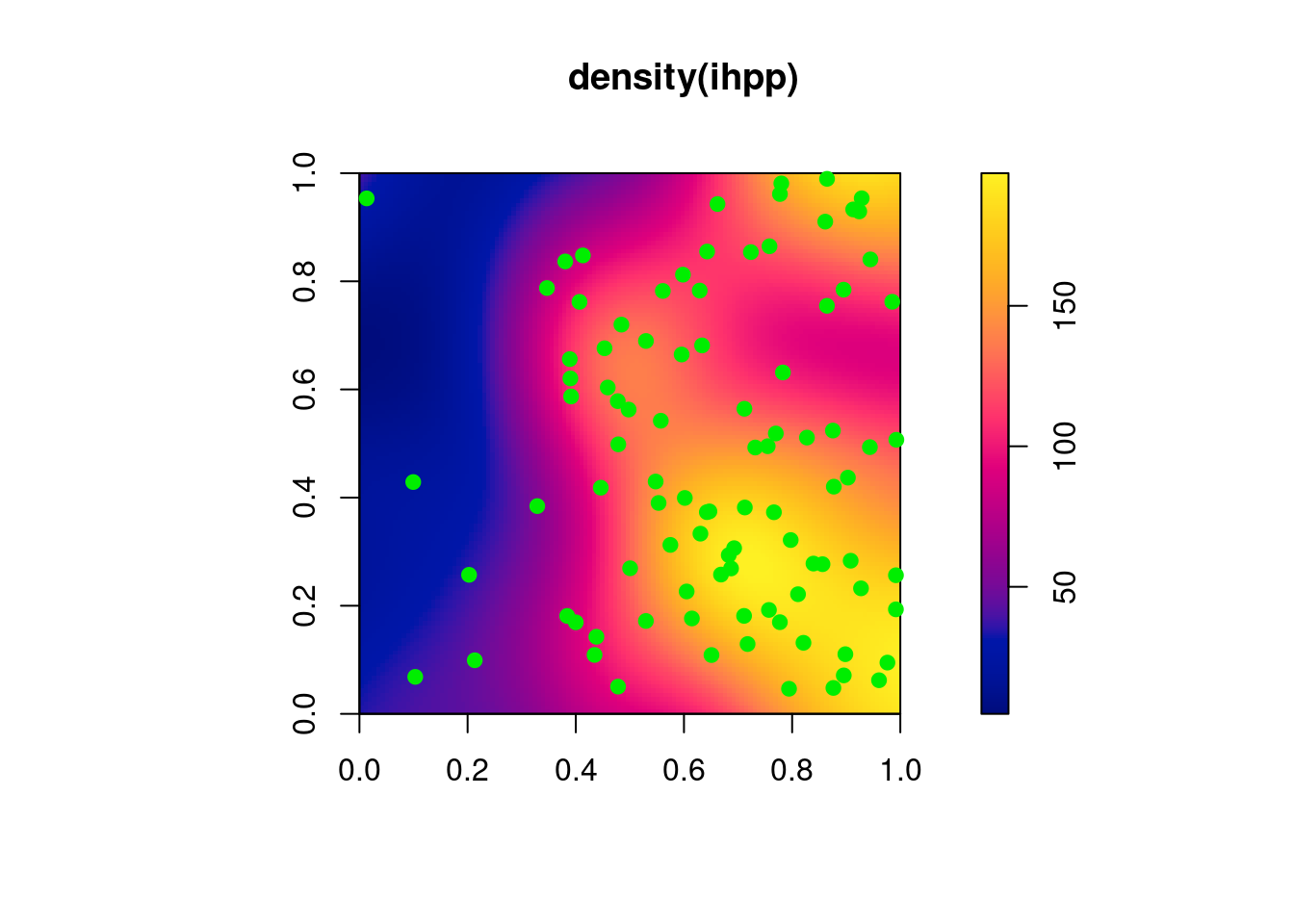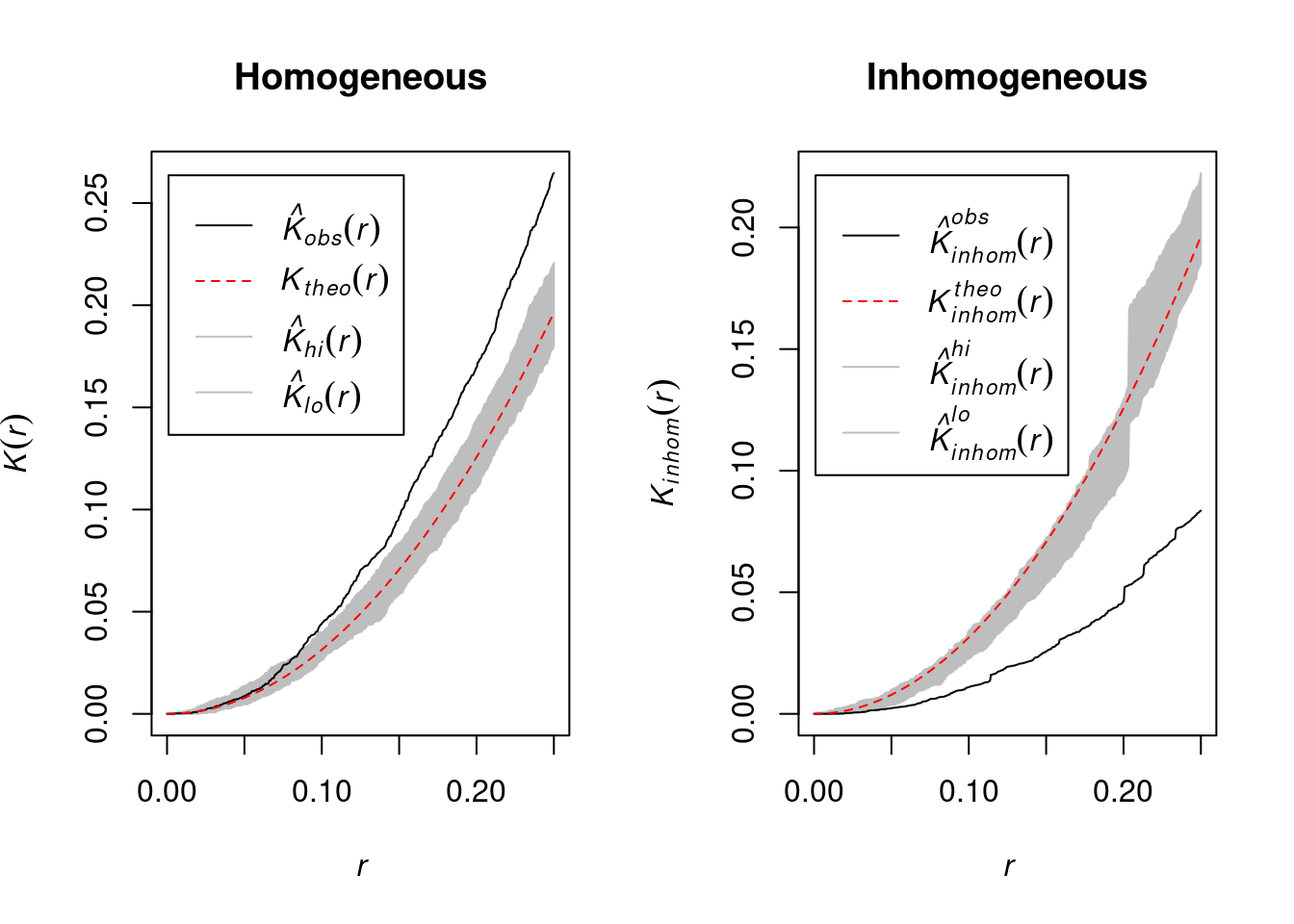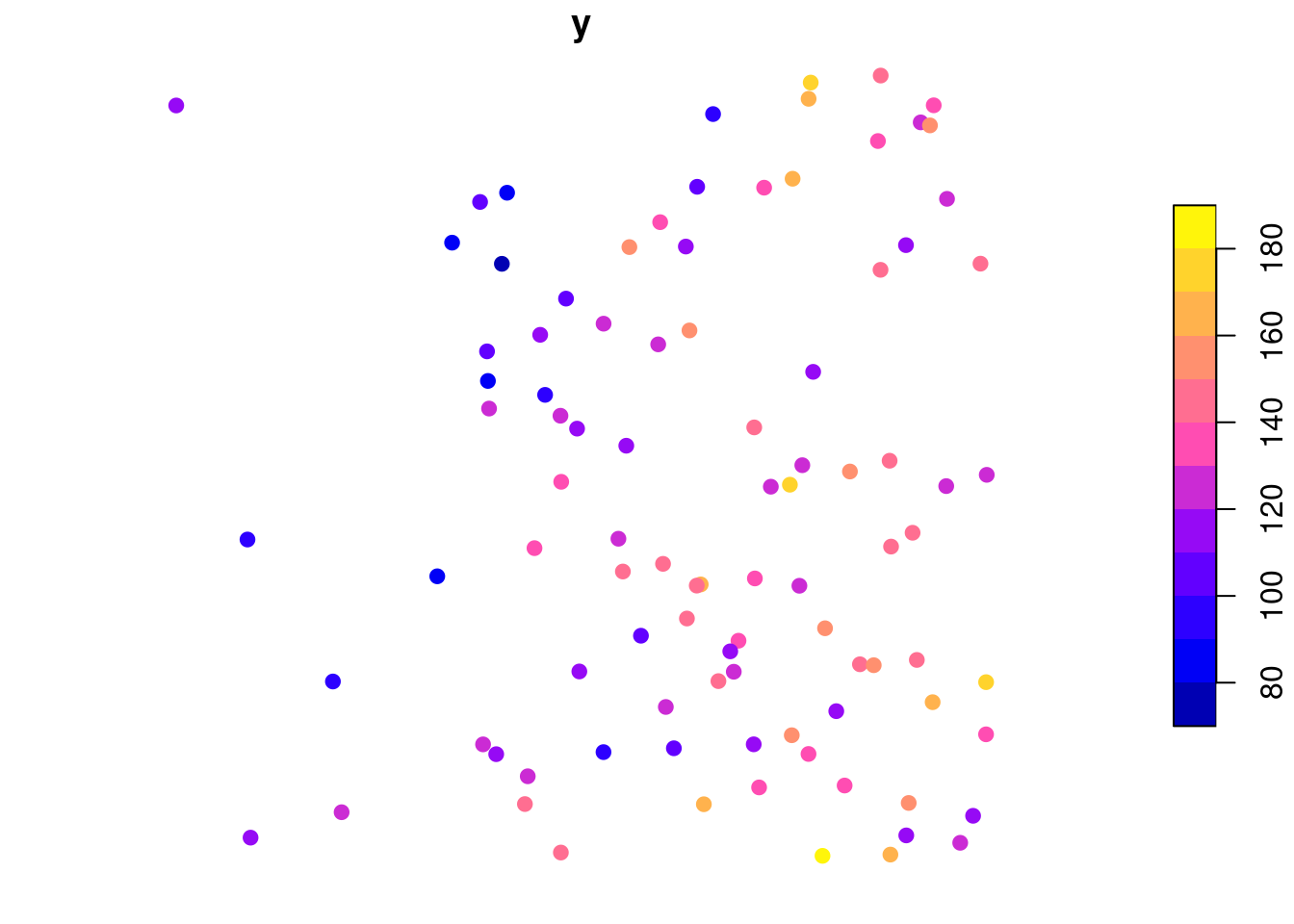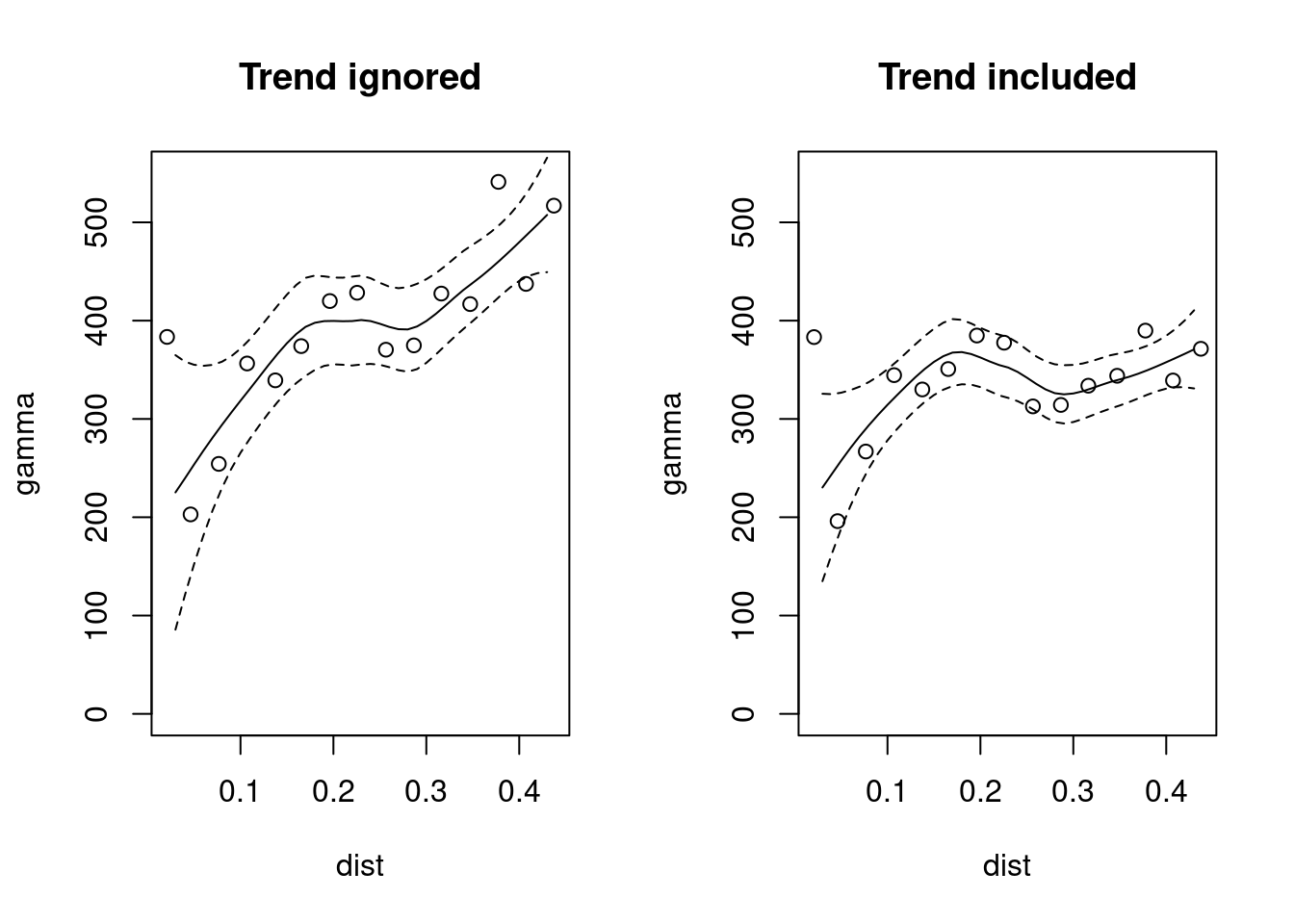### Required current contributed CRAN packages:

spatstat sf gstat spdep tmap spatialreg igraph hglm metafor sp spData RANN

and spDataLarge - install.packages("spDataLarge", repos = "https://nowosad.github.io/drat/", type = "source").

### Script

Script at https://raw.githubusercontent.com/edzer/UseR2019/master/part2.R. Dowload to suitable location and use as basis.

## Is spatial autocorrelation just poor data collection design and/or model mis-specification?

• Spatial, time series, and spatio-temporal data break the fundamental rule of independence of observations (social networks do too)

• Often, we are not sampling from a known population to get observations, so caution is needed for inference

• Often again, the observation units or entities are not chosen by us, but by others

• Designing spatial samples has become a less important part of the field than previously (Ripley 1981; Müller 2007)

### Spatial modelling: why?

• If we want to detect and classify patterns (ML), infer about covariates, or interpolate from a fitted model, we need models that take account of the possible interdependency of spatial, time series and spatio-temporal observations

• Here we are focussing on the spatial case; time differs in having a direction of flow, and spatio-temporal processes are necessarily more complex, especially when non-separable

• We will not look at machine learning issues, although the partition into training and test sets raises important spatial questions for tuning (Schratz et al. 2019)

### Spatial modelling: which kinds?

• Spatial point processes are most closely tied to the relative positions of the observations, but may accommodate inhomegeneities

• Geostatistics is concerned with interpolating values of a variable of interest at unobserved locations, and the relative positions of the observations contribute through the modelling a function of differences in observed values of that variable between observations at increasing distances from each other

• Disease mapping, spatial econometrics/regression and the application of generalized linear mixed models (GLMM, GAMM) in for example ecology are more interested in inferences about the spatial processes in play and the included covariates; some approaches may use distance based spatial correlation functions, others use graph or lattice neighbourhoods

### When is a “spatial” process actually induced by the analyst

• Sometimes when we use spatial data, we jump to spatial statistical methods because of the widely cited first law of geography, that nearby observations are more likely to be similar than those further away

• But maybe what we see as clustering, patchiness, pattern, that looks spatial is actually mis-specification, such as a missing covariate, and/or inappropriate functional form, and/or including variables acting at different scales, and/or consequences of suboptimal bounding of tesselated observations …

• Here, we’ll first look at the consequences of treating inhomogeneous points as homogeneous (the intensity trends upwards with x)

• Then we’ll use those points to see what happens in adding a similar trend to a random variable; empirical variograms are constructed ignoring and including the trend, and tests for global and local spatial autocorrelation are calculated

### Spatial point processes

We can start by using spatstat (Baddeley, Rubak, and Turner 2015) to generate completely spatially random (CSR) points with intensity increasing with x to introduce trend inhomogeneity in a unit square (note the use of set.seed():

suppressPackageStartupMessages(library(spatstat))
intenfun <- function(x, y) 200 * x
set.seed(1)
(ihpp <- rpoispp(intenfun, lmax = 200))
## Planar point pattern: 95 points
## window: rectangle = [0, 1] x [0, 1] units
plot(density(ihpp), axes=TRUE)
points(ihpp, col="green2", pch=19)We can use $$\hat{K}$$ tests ignoring and including inhomogeneity to adapt the test to the underlying data generation process. The homogeneous test examines the divergence from a theoretical CSR at distance bins, the inhomogeneous tries to guess the kind of patterning in the relative positions of the point observations. If we ignore the inhomogeneity, we find significant clustering at most distances, with the opposite finding when using the test attempting to accommodate inhomogeneity:

opar <- par(mfrow=c(1,2))
plot(envelope(ihpp, Kest, verbose=FALSE), main="Homogeneous")
plot(envelope(ihpp, Kinhom, verbose=FALSE), main="Inhomogeneous")par(opar)

### Adding a y trend variable

Coercing the spatstat "ppp" object to an sf "sf" object is trivial, but first adds the window of the point process, which we need to drop by retaining only those labelled "point". Then we take the x coordinate and use it to create y, which trends with x; the DGP is not very noisy.

library(sf)
## Linking to GEOS 3.7.2, GDAL 3.0.0, PROJ 6.1.1
#st_as_sf(ihpp) %>% dplyr::filter(label == "point") -> sf_ihpp
st_as_sf(ihpp) ->.; .[.$label == "point",] -> sf_ihpp crds <- st_coordinates(sf_ihpp) sf_ihpp$x <- crds[,1]
sf_ihpp$y <- 100 + 50 * sf_ihpp$x + 20 * rnorm(nrow(sf_ihpp))
plot(sf_ihpp[,"y"], pch=19)### Variogram model

Variograms for models ignoring (y ~ 1) and including (y ~ x) the trend; if we ignore the trend, we find spurious relationships, shown by loess() fits

suppressPackageStartupMessages(library(gstat))
vg0 <- variogram(y ~ 1, sf_ihpp)
vg1 <- variogram(y ~ x, sf_ihpp)
opar <- par(mfrow=c(1,2))
plot(gamma ~ dist, vg0, ylim=c(0,550), main="Trend ignored")
s <- seq(0, 0.5, 0.01)
p0 <- predict(loess(gamma ~ dist, vg0, weights=np), newdata=data.frame(dist=s), se=TRUE)
lines(s, p0$fit) lines(s, p0$fit-2*p0$se.fit, lty=2) lines(s, p0$fit+2*p0$se.fit, lty=2) plot(gamma ~ dist, vg1, ylim=c(0,550), main="Trend included") p1 <- predict(loess(gamma ~ dist, vg1, weights=np), newdata=data.frame(dist=s), se=TRUE) lines(s, p1$fit)
lines(s, p1$fit-2*p1$se.fit, lty=2)
lines(s, p1$fit+2*p1$se.fit, lty=2)par(opar)

### Spatial autocorrelation

Going on from a continuous to a discrete treatment of space, we can use functions in spdep to define neighbours and then test for global and local spatial autocorrelation. Making first a triangulated neighbour objects, we can thin out improbable neighbours lying too far from one another using a sphere of influence graph to make a symmetric neighbour object:

suppressPackageStartupMessages(library(spdep))
nb_tri <- tri2nb(crds)
(nb_soi <- graph2nb(soi.graph(nb_tri, crds), sym=TRUE))
## Neighbour list object:
## Number of regions: 95
## Number of nonzero links: 302
## Percentage nonzero weights: 3.34626
## Average number of links: 3.178947

The sphere of influence graph generates three subgraphs; a bit unfortunate, but we can live with that (for now):

plot(nb_soi, crds)